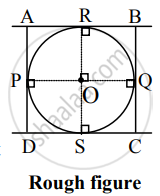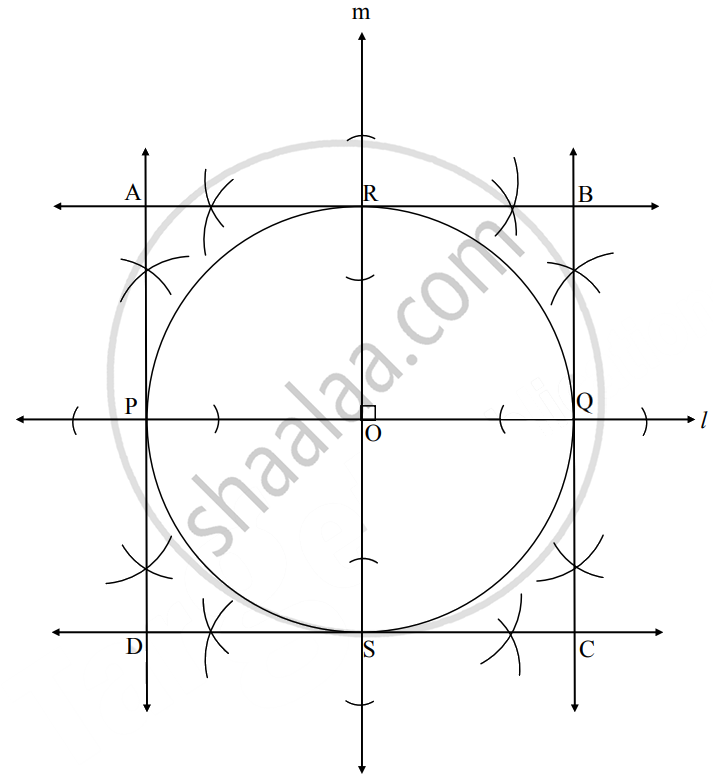# Draw a circle with radius 3 cm. Construct a square such that each of its side will touch the circle from outside - Geometry

Diagram

Draw a circle with radius 3 cm. Construct a square such that each of its side will touch the circle from outside

#### SolutionAnalysis:

In given figure, O is the centre of the circle with radius 3 cm, seg PQ and RS are the diameters of the circle, perpendicular to each other.

seg AB, seg BC, seg CD, seg DA are tangents to the circle at points R, Q, S, P respectively.

In ▢APOR, ∠R = ∠P = 90°     ......[Tangent theorem]

∠O = 90°     ......[∵ diameter RS ⊥ diameter PQ]

∴ ∠A = 90°    ......[Remaning angle of ▢APOR]

Also seg OR = seg OP    ......…[Radii of same circle]

∴ ▢APOR is a square    ......[∵ Each angle is 90° and adjcent sides are congruent]

Similary, ▢AOQB, ▢OSCQ and ▢PDSO are squares.

Also, seg OP = seg OQ = seg OQ = seg OS

∴ ▢APOR, ▢AOQB, ▢OSCQ and ▢PDSO are congruent

∴ ▢ABCD is a square.

∴ To draw square ABCD, we draw two perpendicular diameters and draw tangents at their endpoints.Steps of construction:

1. With Centre O, draw a circle of radius 3 cm.
2. Draw line l passing through O, intersecting circle at points P and Q.
3. Draw line m passing through O, perpendicular to line l, intersecting circle at points R and S.
4. Draw perpendiculars AB and CD to line m at points R and S respectively.
5. Draw perpendiculars AD and BC to line l at points P and Q respectively.
▢ABCD is the required square.
Concept: Construction of a Tangent to the Circle at a Point on the Circle
Is there an error in this question or solution?

Share